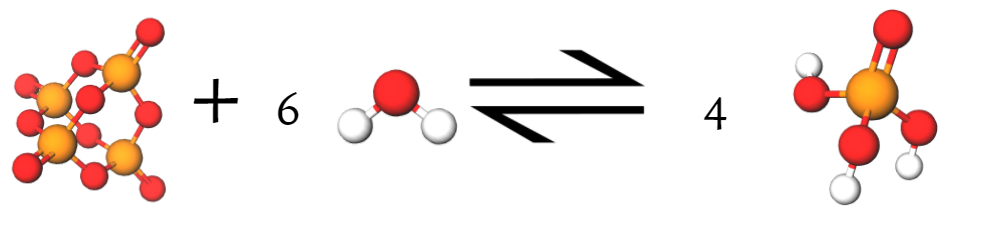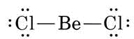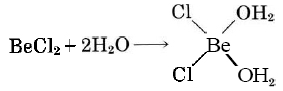# 11.14: Lewis Acids and Bases

Many oxyacids are rather unstable and cannot be isolated in pure form. An example is carbonic acid, H2CO3, which decomposes to water and carbon dioxide:

$\text{H}_2 \text{CO}_3(aq) \rightleftharpoons \text{H}_2\text{O}(l) + \text{CO}_2(g)$

This decomposition process is familiar to us, as it is responsible for the fizzy nature of soda. When the CO2 is dissolved in the soda, it becomes H2CO3, but when the soda is released from high pressure, the decomposition process occurrs rapidly, forming bubbles of CO2 and water.Since it can be made by removing H2O from H2CO3, CO2 is called the acid anhydride of H2CO3. (The term anhydride is derived from anhydrous, meaning “not containing water.”) Acid anhydrides are usually oxides of nonmetallic elements. Some common examples and their corresponding oxyacids are SO2—H2SO3; SO3—H2SO4; P4O10—H3PO4; N2O5—HNO3. Any of these anhydrides increases the hydronium-ion concentration when dissolved in water; for example,

$\text{P}_4\text{O}_{10}(s) + 6\text{H}_2\text{O}(l) \rightarrow 4 \text{H}_3\text{PO}_4(aq)$$\text{H}_3\text{PO}_4(aq) + \text{H}_2\text{O}(l) \rightleftharpoons \text{H}_3\text{O}^+(aq) + \text{H}_2\text{PO}_4^-(aq)$In the Arrhenius sense, then, acid anhydrides are acids, but according to the Brönsted-Lowry definition, they are not acids because they contain no hydrogen.

In 1923, at the same time that the Brönsted-Lowry definition was proposed, G. N. Lewis suggested another definition which includes the acid anhydrides and a number of other substances as acids. According to the Lewis definition, an acid is any species which can accept a lone pair of electrons, and a base is any species which can donate a lone pair of electrons. An acid-base reaction in the Lewis sense involves formation of a coordinate covalent bond (where one atom provides both shared electrons).

The Lewis definition has little effect on the types of molecules we expect to be basic. All the Brönsted-Lowry bases, for example, NH3, O2–, H, contain at least one lone pair. Lewis’ idea does expand the number of acids, though. The proton is not the only species which can form a coordinate covalent bond with a lone pair. Cations of the transition metals, which are strongly hydrated, do the same thing:

\begin{align} & \underset{\text{Lewis acid}}{\text{Cr}^{\text{3+}}}\text{ + }\underset{\text{Lewis base}}{\text{6H}_{\text{2}}\text{O}}\text{ }\to \text{ Cr(H}_{\text{2}}\text{O)}_{\text{6}}^{\text{3+}} \\ & \underset{\text{Lewis acid}}{\text{Cu}^{\text{2+}}}\text{ + }\underset{\text{Lewis base}}{\text{4NH}_{\text{3}}}\text{ }\to \text{ Cu(NH}_{\text{3}}\text{)}_{\text{4}}^{\text{2+}} \\ \end{align}

So can electron deficient compounds such as boron trifluoride:Many Lewis acid-base reactions occur in media other than aqueous solution. The Brönsted-Lowry theory accounts for almost all aqueous acid-base chemistry. Therefore the Brönsted-Lowry concept is most often intended when the words acid or base are used. The Lewis definition is useful when discussing transition-metal ions, however, and is discussed again in the sections on Metals.

Example $$\PageIndex{1}$$ : Lewis Acids and Bases

Identify the Lewis acids and bases in the following list. Write an equation for the combination of each acid with the Lewis base H2O.(a) BeCl2(g); (b) CH3OH; (c) SO2; (d) CF4.

Solution

a) The Lewis diagramshows that Be is electron deficient. Therefore BeCl2(g) is a Lewis acid. Because of the lone pairs on the Cl atoms, BeCl2 can also act as a Lewis base, but Cl is rather electronegative and reluctant to donate electrons, so the Lewis base strength of BeCl2 is less than the Lewis acid strength.b) There are lone pairs on O in CH3OH, and so it can serve as a Lewis base.

c) The S atom in SO2 can accept an extra pair of electrons, and so SO2 is a Lewis acid. The O atoms have lone pairs but are only weakly basic for the same reason as the Cl atoms in part (a).d) Although there are lone pairs on the F atoms, the high electronegativity of F prevents them from being donated to form coordinate covalent bonds. Consequently CF4 has essentially no Lewis-base character.